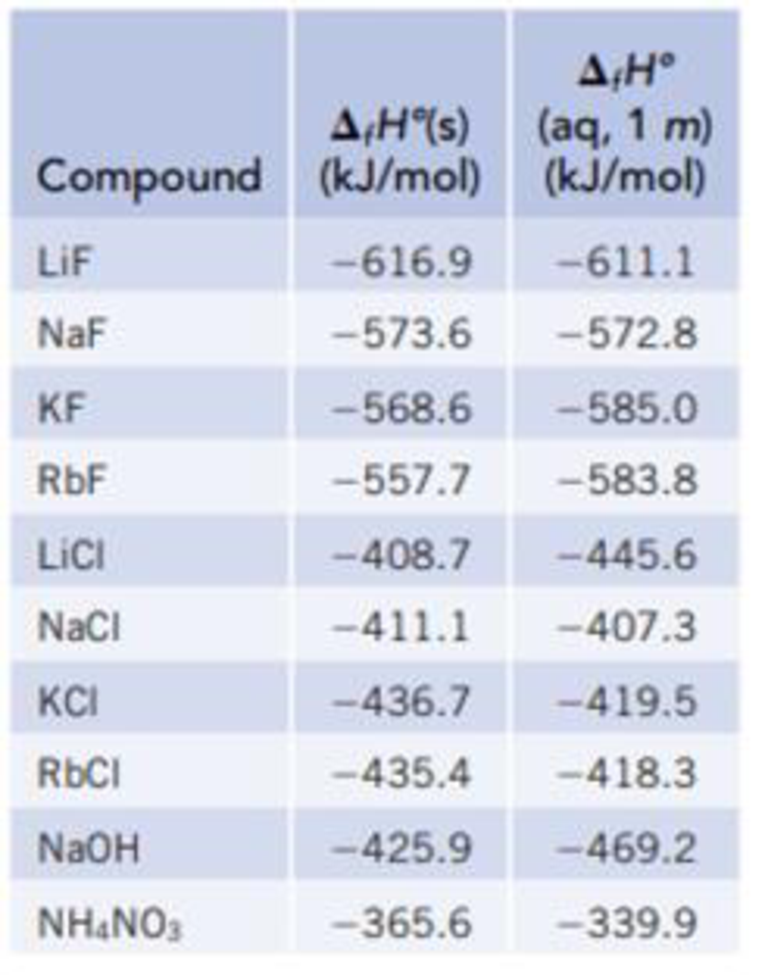Chapter 13, Problem 15PS

Chapter
Section
Textbook Problem

Use the data of Table 13.1 to calculate the enthalpy of solution of LiCl.TABLE 13.1Data for calculating enthalpy of SolutionInterpretation Introduction

Interpretation: The enthalpy of solution of LiCl has to be identified.

Concept introduction:

Enthalpy of solution: In the process, the change in enthalpy while dissolving the solute in the solvent.

ΔsolnHo = [fHo(Product)]-[fHo(Reactant)]

Explanation

Given data:

Enthalpy of formation of LiCl in the solid state = - 408.7 kJ/molEnthalpy of formation of LiCl in the solution    = - 445.6 kJ/mol

• The enthalpy of solution:

Given:LiCl(s)LiCl(aq)ΔsolnHo = [fHo(Product)]-[f

Still sussing out bartleby?

Check out a sample textbook solution.

See a sample solution

The Solution to Your Study Problems

Bartleby provides explanations to thousands of textbook problems written by our experts, many with advanced degrees!

Get Started

Find more solutions based on key concepts